# ISEE Upper Level Math : Operations

## Example Questions

### Example Question #21 : Operations

Simplify the following expression: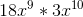Possible Answers: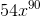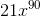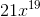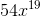Correct answer:Explanation:

Simplify the following expression:To begin, recall that we can multiply the coefficients just like regular integers: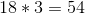To multiply variable of the same exponent, we simply add the exponents: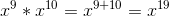Now, put together the two parts and we have our answer:### Example Question #21 : Variables

Simplify the following: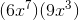Possible Answers: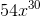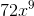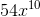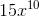Correct answer:Explanation:

Simplify the following:To multiple this out, we need to recall how to multiple variables with exponents, and how to multiply coefficients.

To multiple the coefficients (numbers in front) simply treat them like regular multiplications.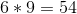So far so good.

To combine our x's, we will add the exponents. Recall that multiplying variables means you add the exponents.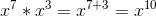Now, put those two together to get:### Example Question #1 : How To Divide Variables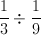Possible Answers: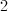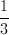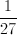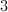Correct answer:Explanation:

Never divide fractions!  Simply flip the fraction that follows the division symbol, and then multiply it by the first fraction.  So this expression becomes: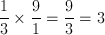### Example Question #22 : Variables

Divide: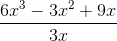Possible Answers: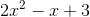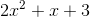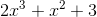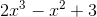Correct answer:Explanation: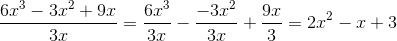### Example Question #3 : How To Divide Variables

Simplify: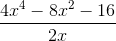Possible Answers: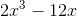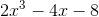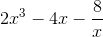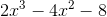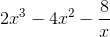Correct answer:Explanation: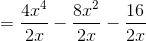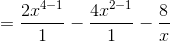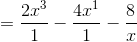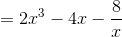### Example Question #23 : Variables

If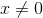, divide: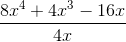Possible Answers: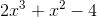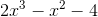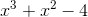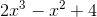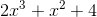Correct answer:Explanation: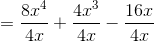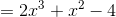### Example Question #5 : How To Divide Variables

Divide: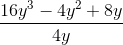Possible Answers: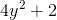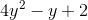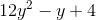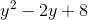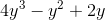Correct answer:Explanation:

In this division problem, you can simplify first the coefficients, then the variables.

Each of the coefficients in the numerator is divisible by the coefficient in the denominator, allowing you to divide out and cancel the 4 in the denominator: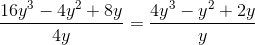Finally, you can simplify the varaibles. Remember that when simplifying variables in a fraction (division problem), you subtract the numerator variable's exponent by the denominator variable's exponent. You can do this with each term in this problem, because each term in the numerator has at least a: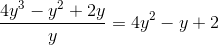### Example Question #6 : How To Divide Variables

Divide: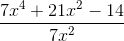Possible Answers: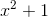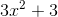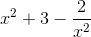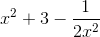Correct answer:Explanation:

In this division problem, you can simplify first the coefficients, then the variables.

Each of the coefficients in the numerator is divisible by the coefficient in the denominator, allowing you to divide out and cancel the 7 in the denominator: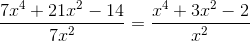Finally, you can simplify the varaibles. Remember that when simplifying variables in a fraction (division problem), you subtract the numerator variable's exponent by the denominator variable's exponent.

You can only do this with the first two terms in the numerator, since the final term does not have a variable. Instead, the final term will keepin the denominator: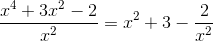### Example Question #7 : How To Divide Variables

Divide: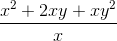Possible Answers: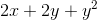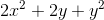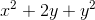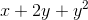Correct answer:Explanation: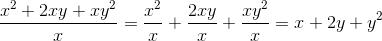### Example Question #8 : How To Divide Variables

Which of the following is a factor of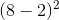?

Possible Answers:Correct answer:Explanation:

The first step to solving this question is to reduce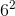The only number listed that is a factor of 36 is 18, given that 2 times 18 is 36. Therefore, 18 is the correct answer.

### All ISEE Upper Level Math Resources